# 简介

现代数字传输技术有许多种，例如MSK , GMSK , OFDM, 扩频技术，但是其中OFDM成功地应用于各种重要的通信系统，例如，DAB与DVB，3G/4G与LTE无线通信系统等。所以本篇文章将着重讲解OFDM流程和原理，以及对多径衰落信道下OFDM的MATLAB实现。

1.1 正弦函数的正交性

1.2 DFT和IDFT

1.3 信号的频域形状

2.1 利用正交的复载波传输信息（正交用在哪？）

2.2 OFDM的原理图

2.2.1 串并变换

2.2.3 将符号调制到正交的子载波

2.2.3 Multiplexing/复用（信号合成）

2.2.4 接收端进行Demultiplexing（信号分解）

2.2.5 接收端进行相干解调

2.2 利用IFFT和FFT进行调制和解调

2.3 循环前缀 （Cyclic Prefix）

2.3.1 ISI 从哪来

2.3.2 如何消除ISI

3.1 仿真的系统（理想信道估计）

3.2 代码

# 一、前置知识

OFDM是一个相比起基本数字调制来讲更为复杂的数字调制技术，我们学习它之前需要足够的前置知识。

## 1.1 正弦函数的正交性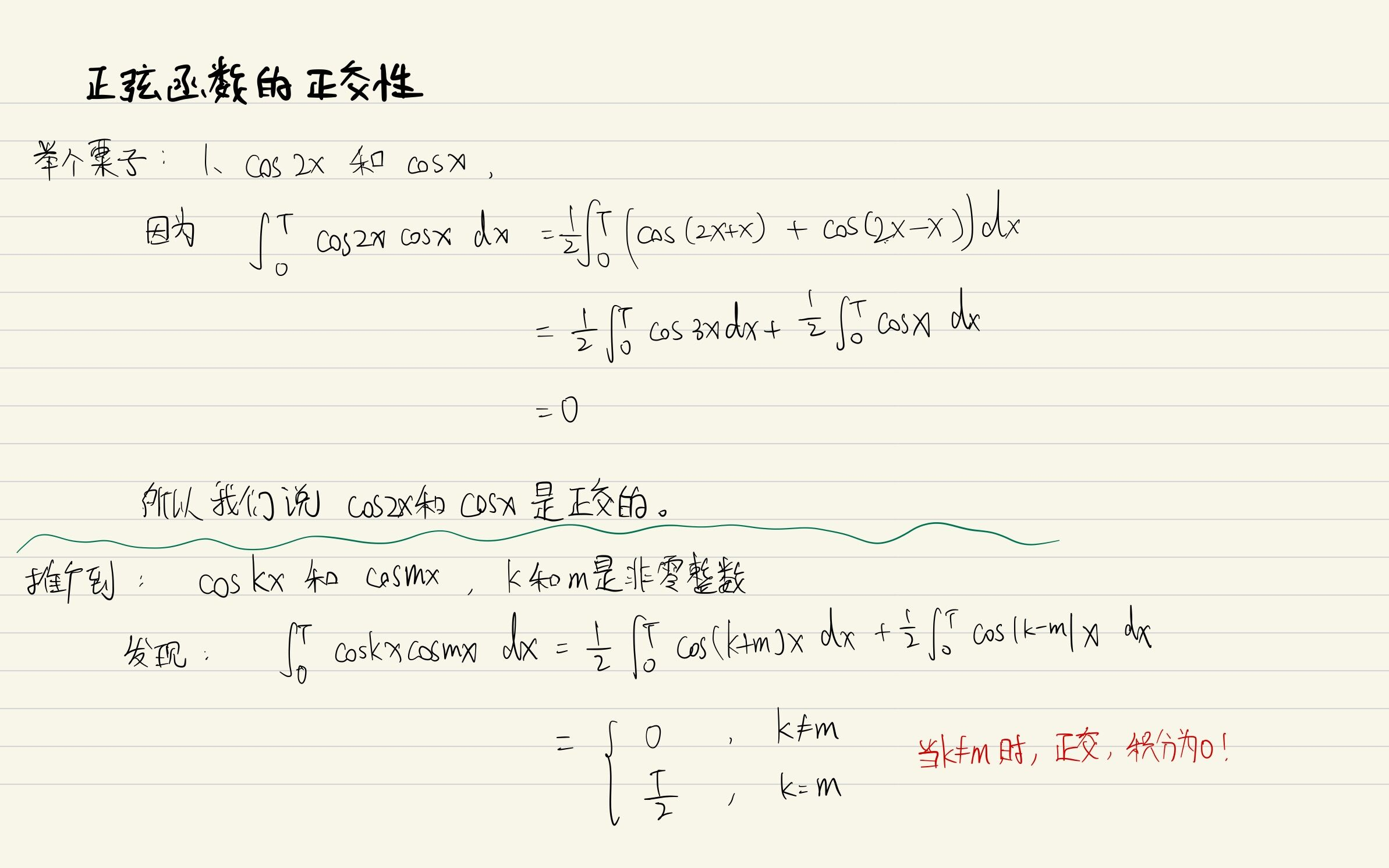通过举例可以看到，正弦函数具有正交性，这样的好处将在接收端进行体现，因为你可以用积分手段去 过滤掉与某一频率的载波正交的其他载波（因为积分为0）。

## 1.2 DFT和IDFT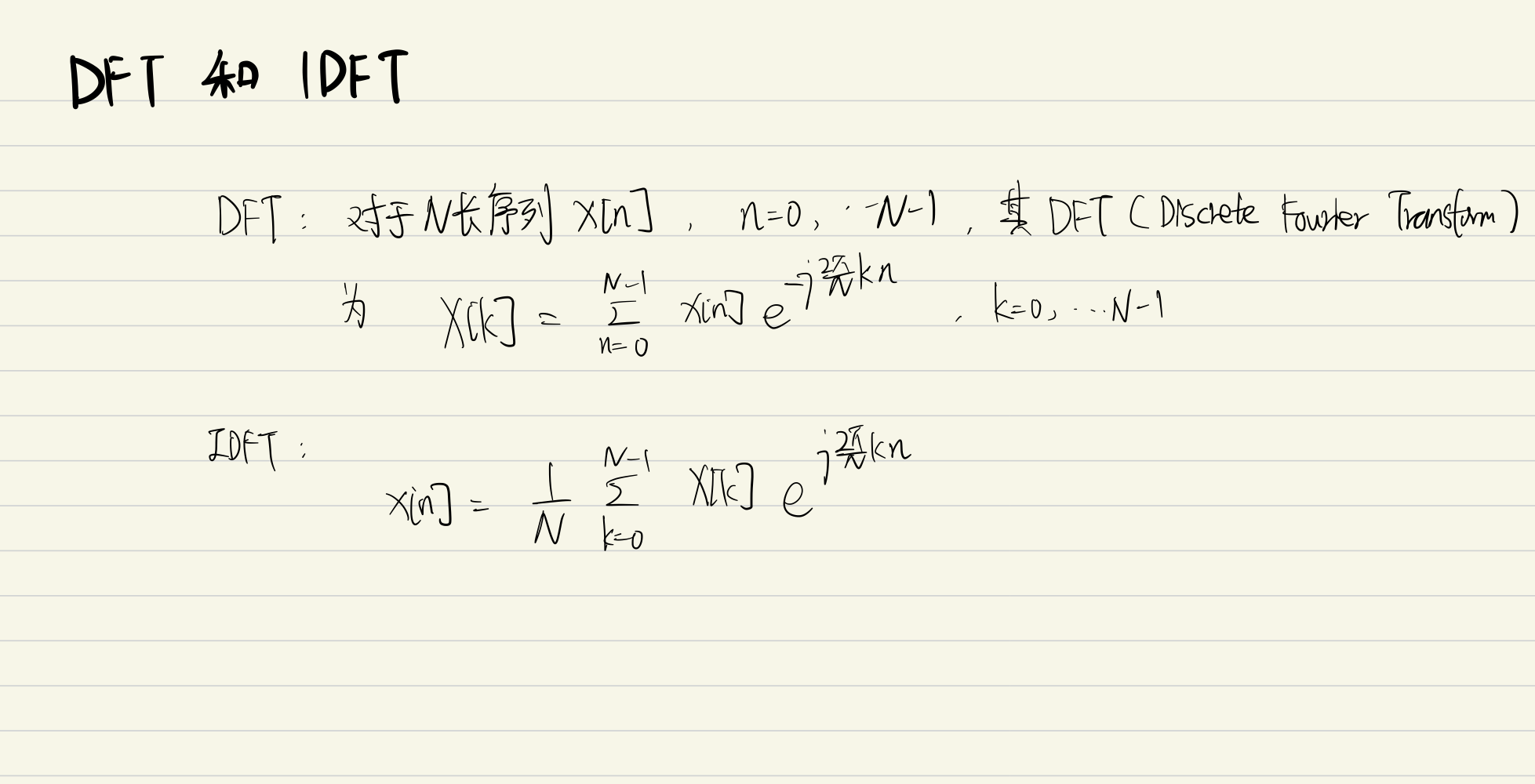记住DFT和IDFT的公式模样，这会是解答为什么OFDM采用IFFT调制信号的关键！

## 1.3 信号的频域形状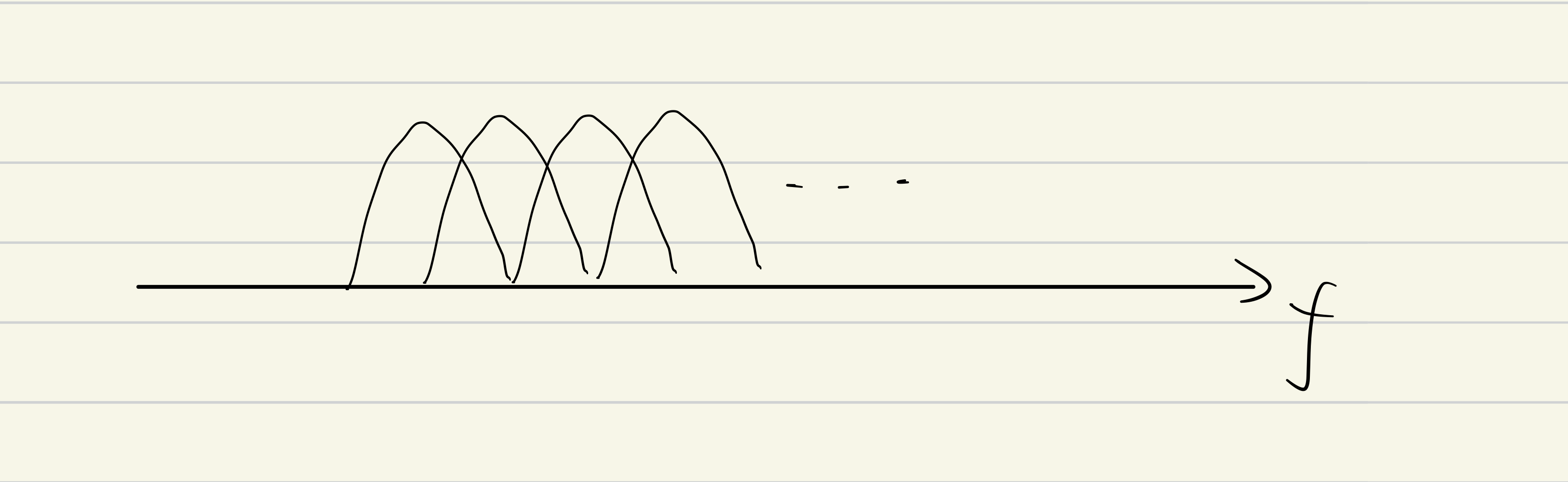为啥明明交叠了也是正交的呢？为什么是这么多拱门状的频域响应呢？我们不慌忙推导正交性，首先关注为什么信号的频率响应是拱门状。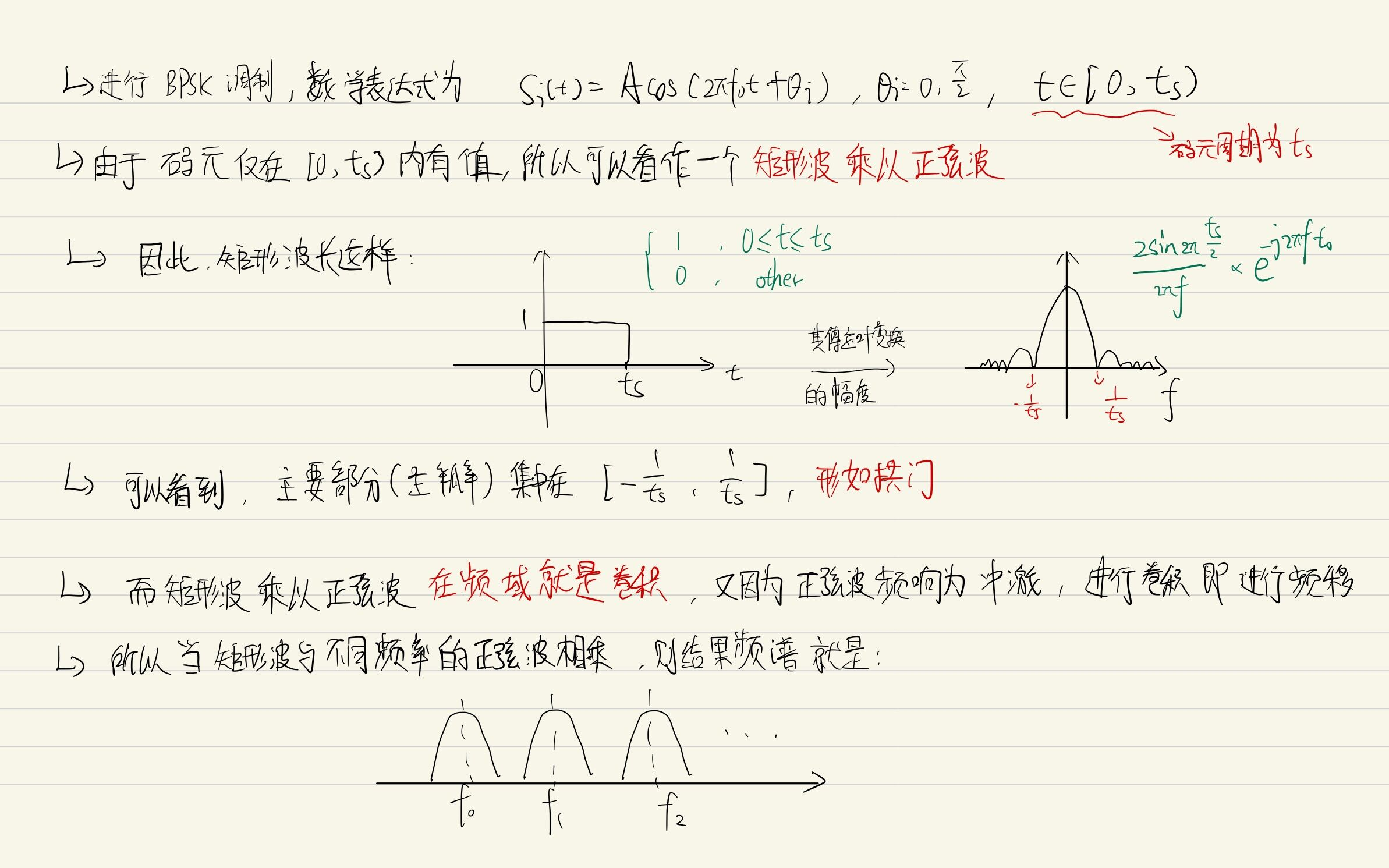从上推导我们得到以下很重要的性质：

1. 有限制码元周期为 Ts 且频率分量为  的调制符号，其频谱就是 拱门状的频率响应进行  的平移。

2. 其主瓣的宽度就是  , 集中在

# 二、ODFM思想（Orthogonal Frequency Division Multiplexing）

## 2.1 利用正交的复载波传输信息（正交用在哪？）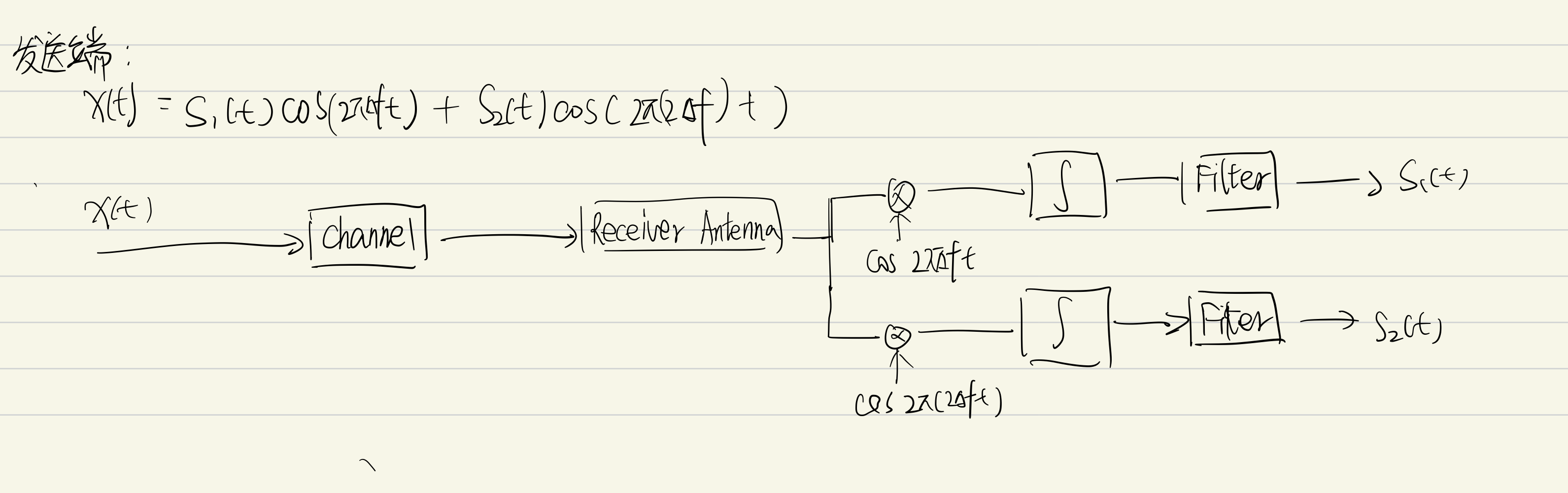那么试想，我用很多个这样的正交复载波去分别传送信息，在接收端都能通过这种手段解调，那不就很方便吗！（后面也将说明其很大程度地节约了频谱资源）

## 2.2 OFDM的原理图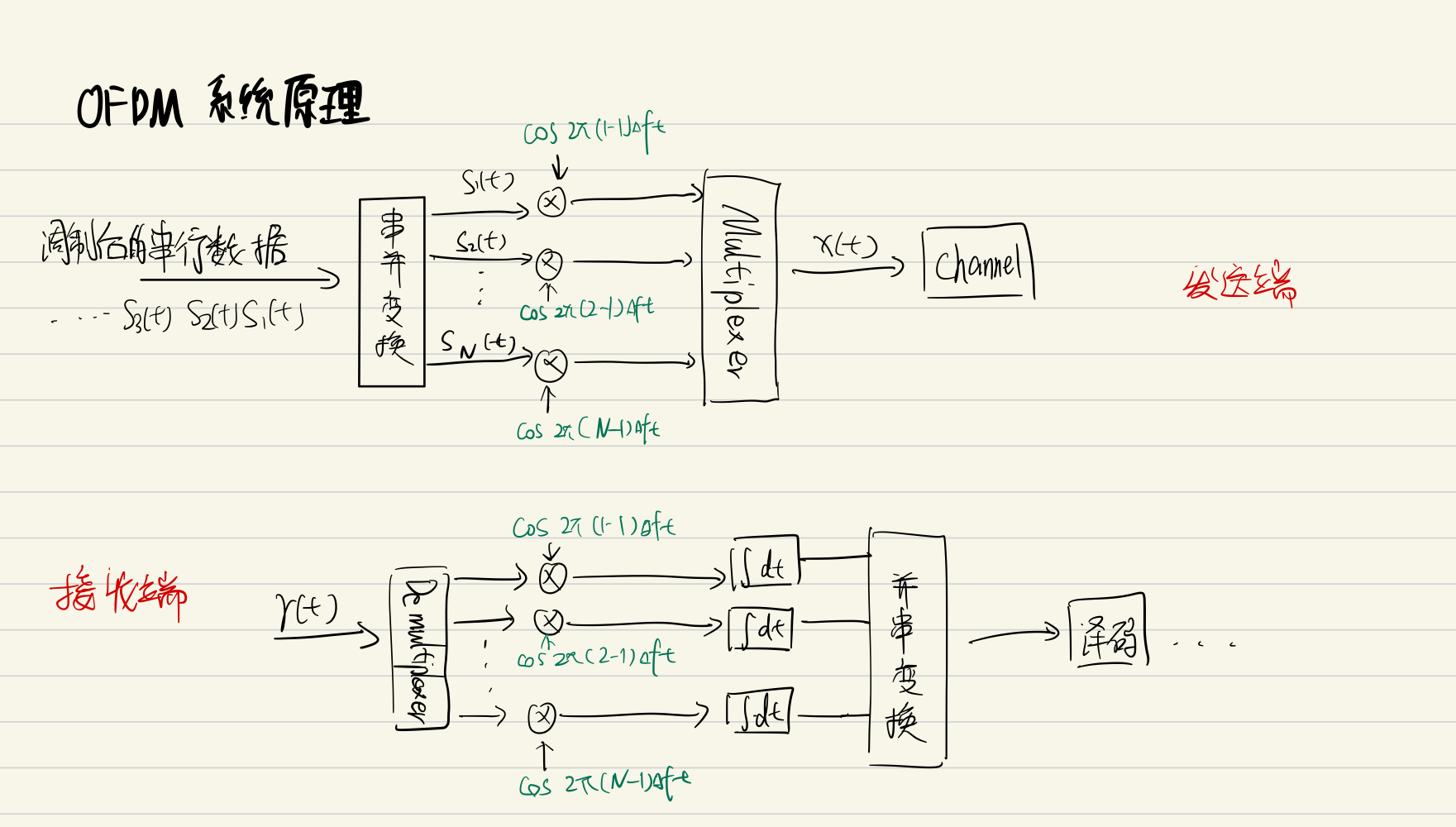### 2.2.3 将符号调制到正交的子载波

1.

2.

所以你会发现，OFDM的频谱长这样：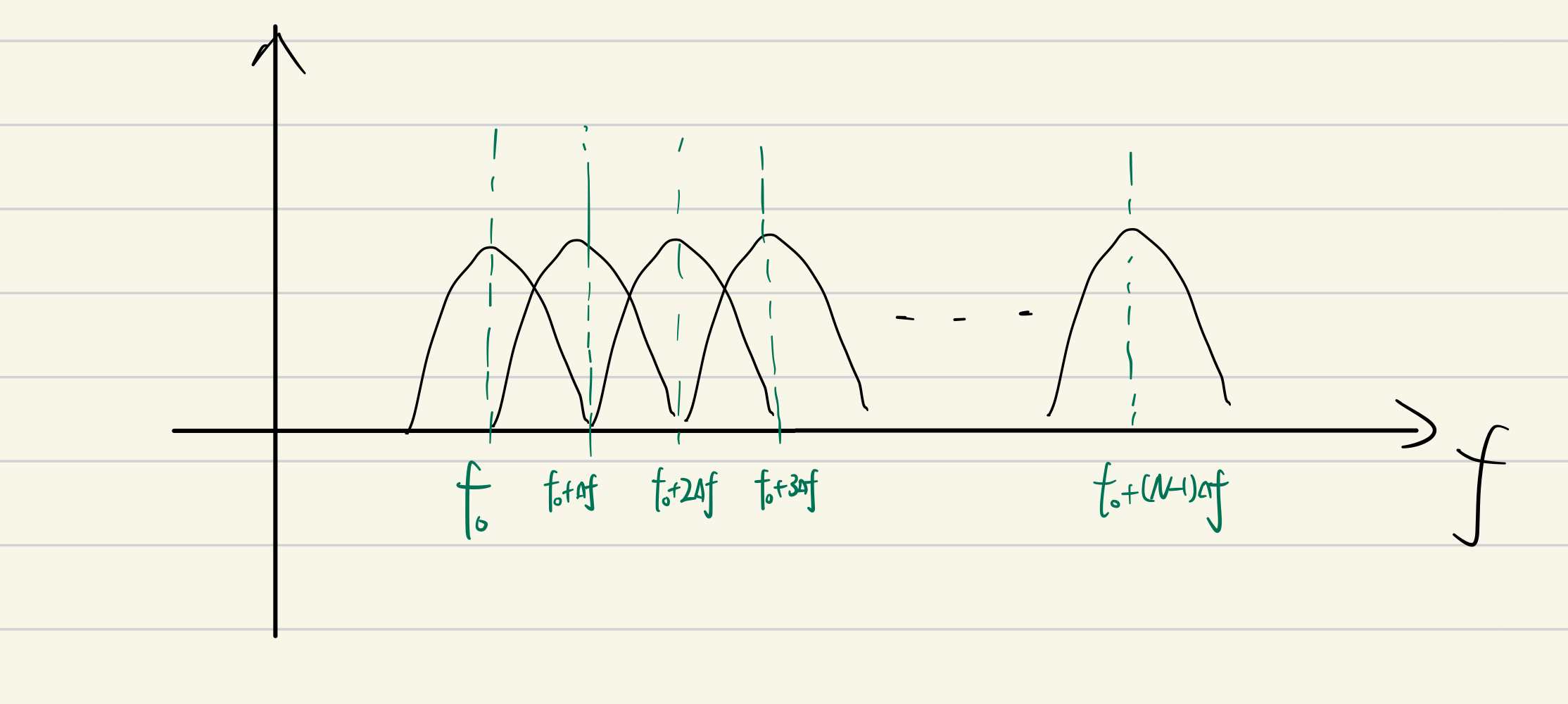## 2.2 利用IFFT和FFT进行调制和解调

所以我们可以用非常便捷的IFFT进行ODFM调制，而在接收端，我们进行FFT即可复原信号。注意了，这里的IFFT和FFT的计算都由DSP处理器计算

## 2.3 循环前缀 （Cyclic Prefix）

### 2.3.1 ISI 从哪来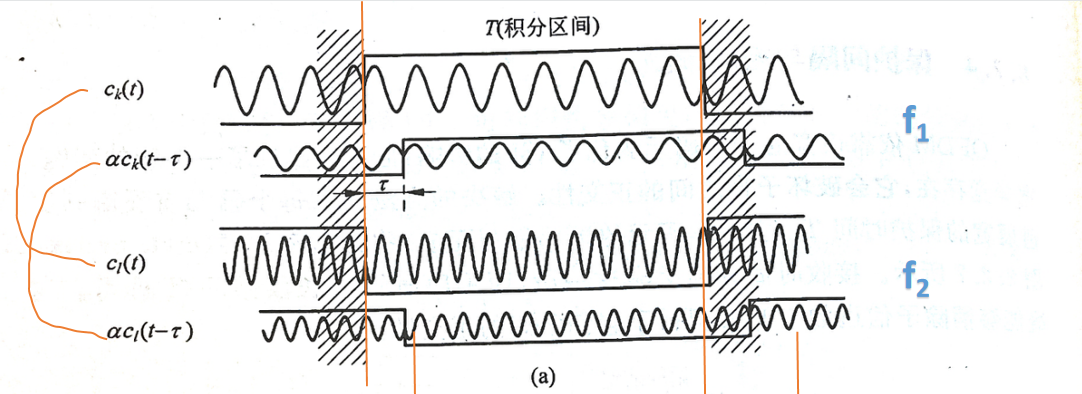只关注T（积分区间），可以看到两个频率的无时延路径（第一行和第三行），在积分区间内彼此正交。但是看两个频率的有时延路径（第二行和第四行），由于时延，两个频率不再正交。两个频率不再正交就意味着这两个子载波在接收端无法用相干解调，造成符号之间的干扰，这就是ISI 来的原因。

### 2.3.2 如何消除ISI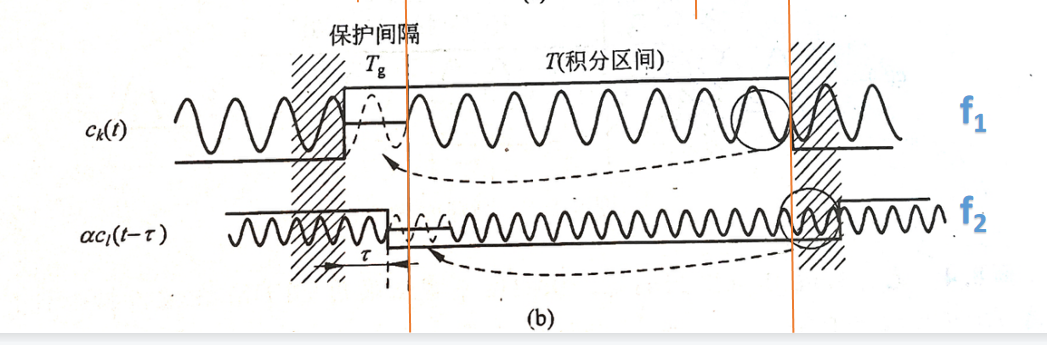可以看到，加入循环前缀后，在T（积分区域），两频率仍然正交。

# 三、OFDM的MATLAB仿真

## 3.1 仿真的系统（理想信道估计）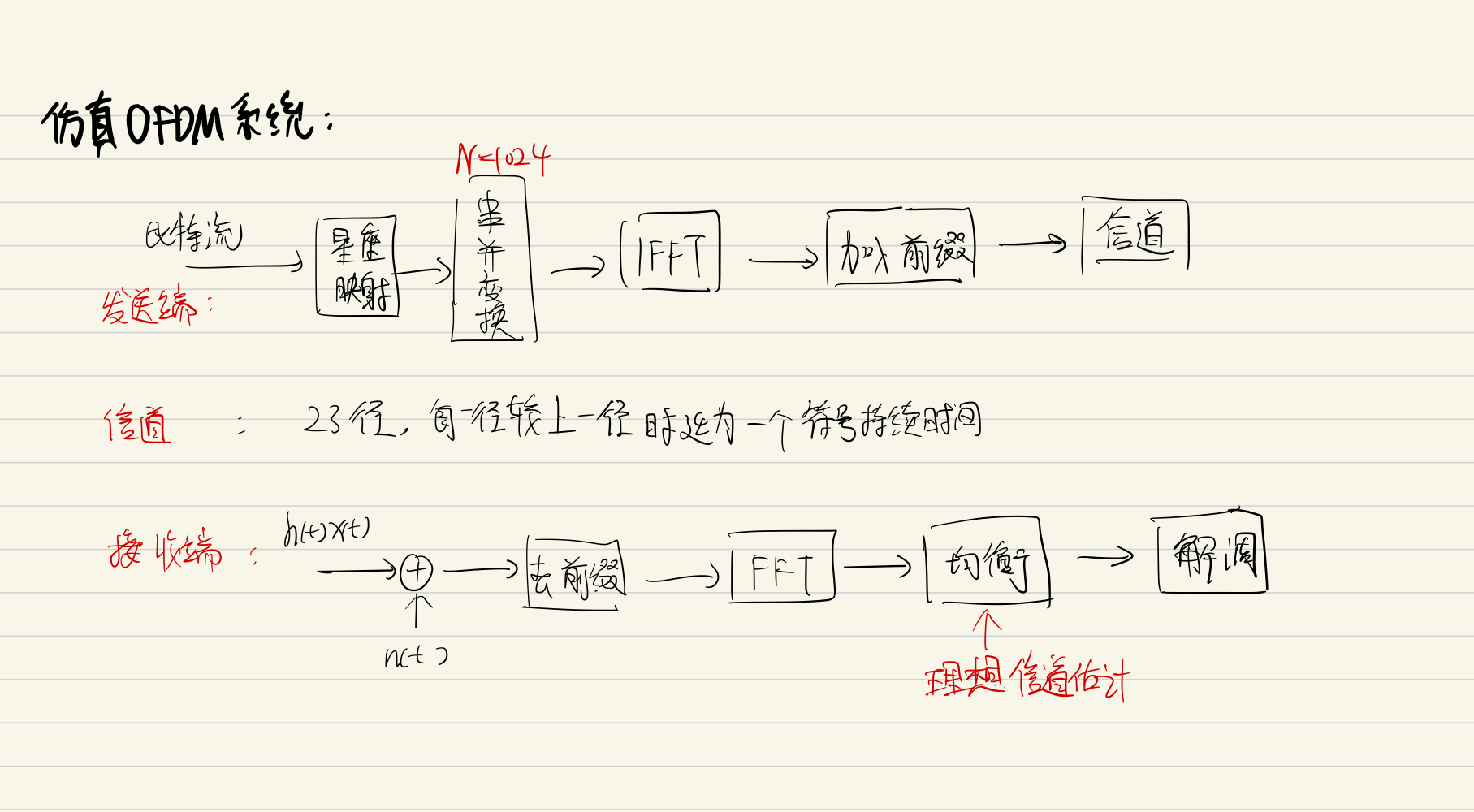## 3.2 代码

function output = OFDM(nos,SNR_dB,H)
%OFDM: This is a function of generation of OFDM symbol and demodulate it
%  BER = OFDM(nos,noise_power_dB,H); where nos is number of symbol; H is
%  dataset of channel
%%
%Generation of OFDM symbols signal
K=1024;  % K number of sub-carriers
nop=23; % Number of paths in channel
tau=1;
tau_max=nop*tau; % The time delay of each path
cp=tau_max+2; % Length of cyclic prefix
OFDM_symbol_overall=[]; % All OFDM Symbol with CP
mes=[]; % Original bit stream - message

for i=1:1:nos
bits=randi([0 1],K,1);  % One OFDM symbol
symbol_bpsk=2*bits-1;  % Modulate bits with BPSK
OFDM_symbol=ifft(symbol_bpsk,K).*sqrt(1024);  % Generation of OFDM symbol and normalization
CP=OFDM_symbol(K-cp+1:K); % Cyclic prefix
OFDM_symbol_cp=[CP;OFDM_symbol];  % Combine CP with OFDM symbol
OFDM_symbol_overall=[OFDM_symbol_overall;OFDM_symbol_cp]; % Combine every OFDM Symbol
mes=[mes;bits];
end

%%
%OFDM signal passed through Multi-paths channel
OFDM_symbols_mp=zeros(nos*(K+cp)+(nop-1)*tau,nop); % OFDM symbols passing through multi-paths channel
for i=1:23
H_part=H( ((i-1)*tau+1) :(nos*(K+cp)+tau*(i-1)),i); % truncate partial of H corresponding to the time delay
OFDM_symbols_mp( ((i-1)*tau+1) :(nos*(K+cp)+(i-1)*tau),i)=OFDM_symbol_overall.*H_part;
end

%%
N0 = 1/10^(SNR_dB/10); % Noise power
noise = sqrt(N0/2)*(randn(1,nos*(K+cp))+1i*randn(1,nos*(K+cp))); % AWGN Noise

%%
% Receive & Normalization & Removing Cyclic prefix
OFDM_signal_rec=sum(OFDM_symbols_mp,2); % Multi-paths Combination
OFDM_signal_rec=OFDM_signal_rec(1:nos*(K+cp),1); % Truncation since the receiver only demodulates specific length of signal
OFDM_signal_rec=OFDM_signal_rec+noise';

rec_nr=zeros(K*nos,1); % Remove the cyclic prefix
for i=1:1:nos
rec_nr( (i-1)*K+1 : i*K )=rec_n(( (i-1) * (K+cp)+cp+1 ): (i*(K+cp)) ,1);
end

%%
%Ideal Channel Estimation - Indicates that we've already known the channal
%Equalizatin

rec_sig_e=zeros(nos*K,1);

for j=1:1:nos
ICE=zeros(K,1);
for i=1:1:nop
H_cor=H( (((j-1)*(K+cp)+cp+1)+(i-1)*tau) : (j*(K+cp)+(i-1)*tau) ,i);
ICE(i)=mean(H_cor);
end
ICE_F=fft(ICE,K);  % The frequence domain of estimated channel
rec_sig_e( ((j-1)*K+1):j*K ,1 )=fft(rec_nr( ((j-1)*K+1):j*K ,1 ),K)./ICE_F;
end

%%
%Detection
x_e=real(rec_sig_e)>0;

%%
%BER
BER=sum(abs(x_e-mes))/(nos*K);
output=BER;
%{
disp(['Information: ']);
disp(['1.The number of OFDM symbols is ',num2str(nos)]);
disp(['2.The S/N in dB is ',num2str(SNR_dB)]);
disp(['3.The bit error rate is ',num2str(BER)]);
%}
end

3.3 OFDM系统（非理想信道估计）

THE END